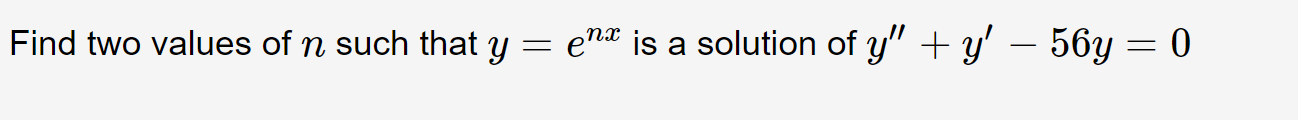# ena is a solution of y" + y' – 56y = 0Find two values of n such that y =

Question
2 viewshelp_outlineImage Transcriptioncloseena is a solution of y" + y' – 56y = 0 Find two values of n such that y = fullscreen
check_circle

star
star
star
star
star
1 Rating
Step 1

y=enx is a solution of the equation y’’+y’-56y=0. So, it must satisfy the equation.

Step 2

Find y’.

Step 3

Find y’&...

### Want to see the full answer?

See Solution

#### Want to see this answer and more?

Solutions are written by subject experts who are available 24/7. Questions are typically answered within 1 hour.*

See Solution
*Response times may vary by subject and question.
Tagged in

### Math